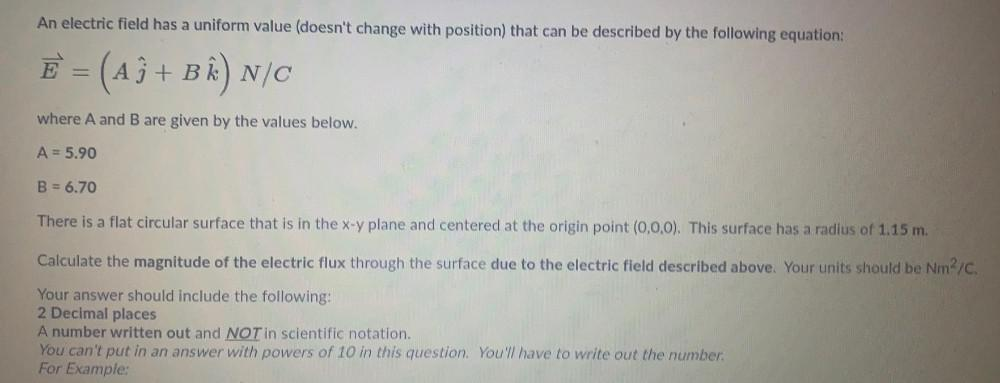Question:

# An electric field has a uniform value (doesn't change with position) that can be described by the following equation: E = (A3+ BAn electric field has a uniform value (doesn't change with position) that can be described by the following equation: E = (A3+ B^N/C where A and B are given by the values below. A = 5.90 B = 6.70 There is a flat circular surface that is in the x-y plane and centered at the origin point (0,0,0). This surface has a radius of 1.15 m. Calculate the magnitude of the electric flux through the surface due to the electric field described above. Your units should be Nm2/C. Your answer should include the following: 2 Decimal places A number written out and NOT in scientific notation. You can't put in an answer with powers of 10 in this question. You have to write out the number: For Example: